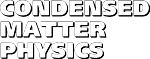Condensed Matter Physics, 2010, vol. 13, No. 1, p. 13002:1-10
DOI:10.5488/CMP.13.13002

Title: Convenient formulae for some integrals in perturbation theory
Author(s):
 D. Henderson (Department of Chemistry and Biochemistry, Brigham Young University, Provo UT 84602)

The free energy and pressure of a fluid, as given by perturbation theory, involve integrals of the hard sphere correlation functions and their density derivatives. In most applications a straightforward procedure would be to obtain these integrals, possibly numerically, using the formulae and computer codes for the hard sphere correlation functions, given previously [Mol. Phys., 2007, 106, 2; Condens. Matter Phys., 2009, 12, 127], followed by numerical differentiation with respect to the density and a possible compounding of errors. More sophisticated methods are given in this paper, which is the second in a planned trilogy, drawn from the author's lecture notes. Three representative model fluids are considered. They are the square-well fluid, the Yukawa fluid, and the Lennard-Jones fluid. Each model fluid is popular for theoretical and engineering calculations and can represent a simple fluid such as argon. With the methods presented here, numerical integration and differentiation are not necessary for the square-well and Yukawa fluids. Numerical integration cannot be easily avoided in the case of the Lennard-Jones fluid. However, numerical differentiation with respect to the density is not required.

Key words: perturbation theory, inverse temperature expansion, compressibility approximations, analytic methods
PACS: 02.30.Qy, 02.30.Rz, 05.20.Jj, 05.70.Ce, 64.30.+t

 Full text [pdf, ps] << List of papers